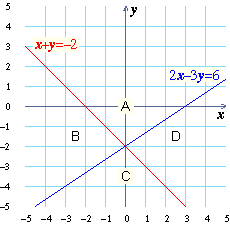# Systems of Linear Inequalities

 A system of inequalities is a list of two or more inequalities that are all required to be true. For example, the pair of inequalities shown to the right is a system of linear inequalities. In this lesson you will learn about solutions to systems of linear inequalities and how to find them by graphing. \$\$\{\,\cl"tight"{\table x, +, 2y, <, -7; 2x, -, 3y, >, 0}\$\$

 We want to solve this system of linear inequalities: \$\$\{\,\cl"tight"{\table y≥-2x-2; y≥-2}\$\$

That is, we want to find the \$(x,y)\$ pairs that are solutions to both inequalities. First, we need to graph the lines \$y=-2x-2\$ and \$y=-2\$. Look at the grids to the left. On the left grid, you are looking at the graph of \$y=-2x-2\$. On the right, you are looking at the graph of \$y=-2\$.

Here is a way to see if the point \$(1,2)\$ is a solution to the first inequality:

\$y≥-2x-2\$ for \$(1,2)\$ ?
\$2≥-2(1)-2\$ ?
\$2≥-2-2\$ ?
\$2≥-4\$ ?
YES

Test each point in the table below in both inequalities. Also indicate which points are solutions to the system of two inequalities (that is, which points are a solution to both the first inequality and the second inequality).

Point Solution to
\$y≥-2x-2\$?
Solution to
\$y≥-2\$?
Solution
to both?
 Click to look at the graphs of the inequalities. Are the blue points that are solutions to \$y≥-2x-2\$ in the shaded region on the left grid?
 Are the blue points that are solutions to \$y≥-2\$ in the shaded region on the right?
 Name the blue points on the grid that are solutions to the entire system (both inequalities).

Click to see \$y≥-2x-2\$ and \$y≥-2\$ graphed together. The dark blue section is the area where the solution sets overlap. The light blue section is the rest of the solution set of \$y≥-2\$ and the pink section is the rest of the solution set of \$y≥-2x-2\$.

 Find on the graph the points you just listed. Are they in the dark blue, the light blue, or the pink section?
 Which colored section of the graph represents the solution set of the entire system of inequalities?
 Now, let’s solve the system of linear inequalities: \$\$\{\,\cl"tight"{\table y≥-4x-2; 3x+4y≤18}\$\$

The red line is the graph of \$y=-4x-2\$ and the blue line is the graph of \$3x+4y=18\$.

For each point in the table below, locate the point on the grid and determine whether it is a solution to each inequality.

PointSolution to
\$y≥-4x-2\$?
Solution to
\$3x+4y≤18\$?
Solution
to both?
 Click to look at the graph of the inequalities. Remember that the dark blue region is the graph of the solution set of the system of linear inequalities. Name the point on the grid that lies in the solution set.
 Does your answer match your answer from the table?
 Now it’s your turn to solve the system of linear inequalities shown here: \$\$\{\,\cl"tight"{\table x,+,y,≥,-2; 2x,-,3y,≤,6}\$\$

The graphs of the lines \$x+y=-2\$ and \$2x-3y=6\$ are given on the grid below. As you can see, the graphs of these two lines divide the grid into four sections, which have been labeled A, B, C, and D.Choose a point in each section of the grid above (A, B, C, and D), and fill in the table below.

SectionPointSolution to
\$x+y≥-2\$?
Solution to
\$2x-3y≤6\$?
Solution
to both?
 Which sections (A, B, C, or D) are solutions to \$x+y≥-2\$?
 Which sections are solutions to \$2x-3y≤6\$?
 Which section is the solution set to the system of linear inequalities?
 Click to graph the two inequalities. Does the dark blue section of the grid to the left correspond to the solution set you just found?
 The top grid to the left shows a system of two inequalities. The bottom grid shows a different system including the inequality \$y